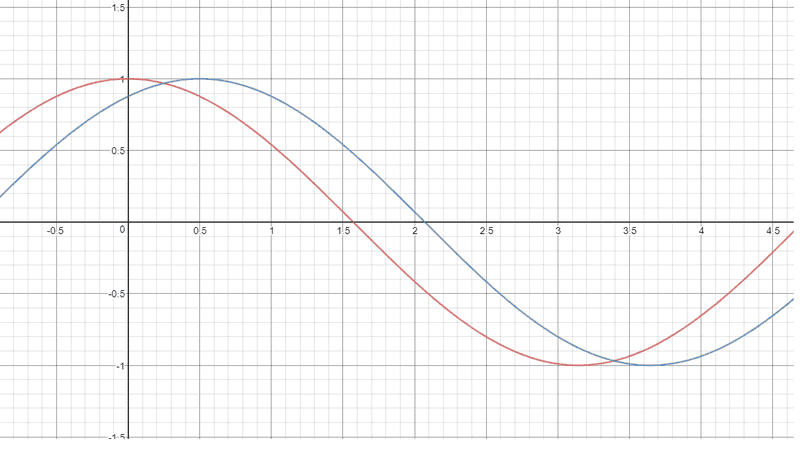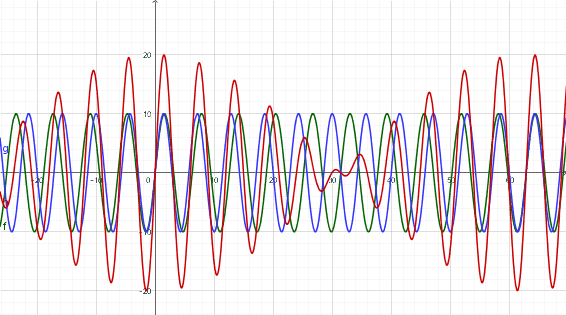# What is meant by natural frequency?

• Sho Kano

#### Sho Kano

An object only undergoes an oscillation when I act on it. If I push a spring, it will have a certain frequency, and if I push a different way, it will have a different one. What is meant by natural frequency? What is meant by resonance?

An object only undergoes an oscillation when I act on it. If I push a spring, it will have a certain frequency, and if I push a different way, it will have a different one. What is meant by natural frequency? What is meant by resonance?
What research have you done on these terms? What have you found out? What part of it do you not understand?

•russ_watters
My book says the natural freq is the freq at which it would oscillate at if suddenly disturbed and left to oscillate freely. But I can clearly push it different ways, which would result in a diff freq. So what is natural freq?

My book says the natural freq is the freq at which it would oscillate at if suddenly disturbed and left to oscillate freely. But I can clearly push it different ways, which would result in a diff freq. So what is natural freq?
You need to explain more, and in precise detail, what you mean by "push it different ways".

But I can clearly push it different ways which would result in a diff freq.
Can you give some examples of the "different ways" which produce different frequencies? (just to make sure we're all talking about the same thing)

[aha, phinds beat me to it]

aka pushing it with a lot of force vs pushing it softly. Pushing it harder intuitively seems to decrease the freq and vice versa, but I don't have an actual spring to test this

aka pushing it with a lot of force vs pushing it softly
That should make exactly ZERO difference in the frequency response to the push, just to the magnitude and duration of the oscillation. I think you are confusing frequency with other characteristics. Have you actually DONE this and observed the results?

•FactChecker
That should make exactly ZERO difference in the frequency response to the push, just to the magnitude and duration of the oscillation. I think you are confusing frequency with other characteristics. Have you actually DONE this and observed the results?
f is related to period, which is related to duration though

aka pushing it with a lot of force vs pushing it softly. Pushing it harder intuitively seems to decrease the freq and vice versa, but I don't have an actual spring to test this
In both cases, it would produce same frequency. As you push harder it develops more potential energy and as per hooke's law,
F=-kx, where x=increased length
So, by pushing harder more force act on the body and the body is pulled towards its mean position faster and will have same no. of oscillating per second.

•Douglas Sunday
aka pushing it with a lot of force vs pushing it softly. Pushing it harder intuitively seems to decrease the freq and vice versa...
That is not correct. A larger force will increase the amplitude but not the frequency.

•Simon Clement
That makes sense; ##k=mw^2##, which means the angular frequency of the spring only depends on the inertia that it has the move around and the k (property of the oscillator) right?

Resonance is when the frequency of the applied outside force matches the frequency of the oscillator, and maximum amplitude occurs. How do you apply a force in a way that "matches the frequency"?

Resonance is when the frequency of the applied outside force matches the frequency of the oscillator, and maximum amplitude occurs. How do you apply a force in a way that "matches the frequency"?
Not really. As long as you put energy into the system, the system may resonate at its natural frequency. The energy you put in does not have to have any frequency that matches the natural frequency. A flag will flap in a steady wind. The reed of a clarinet will vibrate even if you just blow steadily. Same with a whistle.

Sho Kano, are you referring only to systems which move with simple harmonic motion? if so these systems have one natural frequency only. The majority of systems are not simple harmonic and have a range of frequencies eg the notes produced by a stretched string can depend on where you set the string into vibrations.

Last edited:
•Zypheros_Knight
Not really. As long as you put energy into the system, the system may resonate at its natural frequency. The energy you put in does not have to have any frequency that matches the natural frequency. A flag will flap in a steady wind. The reed of a clarinet will vibrate even if you just blow steadily. Same with a whistle.
By resonance he means when the system vibrates with maximum amplitude and that happens when there is a match between forcing and natural frequencies. At resonance maximum energy is drawn from the forcing system

•Zypheros_Knight and FactChecker
Resonance is when the frequency of the applied outside force matches the frequency of the oscillator, and maximum amplitude occurs. How do you apply a force in a way that "matches the frequency"?
There are many different systems. Which ones are you referring to?

By resonance he means when the system vibrates with maximum amplitude and that happens when there is a match between forcing and natural frequencies. At resonance maximum energy is drawn from the forcing system
Good point. Although then I guess it would not depend only on the frequency, but also phase being synchronized.

Last edited:
By resonance he means when the system vibrates with maximum amplitude and that happens when there is a match between forcing and natural frequencies. At resonance maximum energy is drawn from the forcing system

At resonance minimum input of energy is required to sustain the motion ?

There are many different systems. Which ones are you referring to?
I want to look at three situations, a pendulum, a spring, and a glass bottle.

For a pendulum or spring, how would one apply a force to achieve resonance? Apply it "in phase" right? Meaning I push it in the direction of its motion routinely.

Would it matter how hard I push it? All that matters is that the frequency of the driving force (when I push it) is the same as the oscillator's freq right?

It's kinda like adding sinusoidal functions right? You'd want them to be in phase with each other to achieve maximum amplitude when you add them. What I am struggling with is how I would act on a pendulum or spring to achieve resonance.

Last edited:
I want to look at three situations, a pendulum, a spring, and a glass bottle.

For a pendulum or spring, how would one apply a force to achieve resonance? Apply it "in phase" right? Meaning I push it in the direction of its motion routinely.

Would it matter how hard I push it? All that matters is that the frequency of the driving force (when I push it) is the same as the oscillator's freq right?

It's kinda like adding sinusoidal functions right? You'd want them to be in phase with each other to achieve maximum amplitude when you add them. What I am struggling with is how I would act on a pendulum or spring to achieve resonance.
It's not hard. You don't have to apply a force in a sine wave, you can just give it a shove (impulse) at the right time. For a pendulum or spring, when it reaches the top, give it a little shove down. The escape mechanism of a grandfather clock with a pendulum works like that.
I don't know what you mean by a glass bottle. Are you talking about blowing over the top to make it whistle? In that case, you can blow steadily the right way and it will resonate at it's own frequency.

When is the right time for me to give it an impulse? Direction does, but does the magnitude of the impulse matter?

When is the right time for me to give it an impulse?
Any time when it will accelerate the motion instead of opposing it.
Direction does, but does the magnitude of the impulse matter?
Enough energy to overcome damping and friction.

My books says "Thus, if you push a swing at its natural angular frequency, the displacement and the velocity amplitudes will increase to large values, a fact that children learn quickly by trial and error. If you push at other angular frequencies, either higher or lower, the displacement and velocity amplitudes will be smaller." The book means either higher or lower freq which opposes motion right?

So to summarize, to achieve resonance, all you have to do is apply the force at times where it doesn't oppose motion. Applying it at any other frequency will oppose motion. Is this right?

So to summarize, to achieve resonance, all you have to do is apply the force at times where it doesn't oppose motion. Applying it at any other frequency will oppose motion. Is this right?
Applying it at any other frequency will sometimes oppose the motion. As the two frequencies drift in phase with each other, they will sometimes be in phase, reinforcing the motion, and other times out of phase, opposing the motion.

Applying it at any other frequency will sometimes oppose the motion. As the two frequencies drift in phase with each other, they will sometimes be in phase, reinforcing the motion, and other times out of phase, opposing the motion.
Like this graph right? At x=0.25 they are both opposing in motion, then they both descend together, and then oppose againNo. At x=0.25, they are both positive and add together. They oppose in 1.6 < x < 2.0, where one is positive and the other is negative. In general, they add together instead of opposing. They could better synchronized, but not by much.
In the diagram below, the blue and green lines are two different frequencies and the red line is their sum. The red line shows that the blue and green reinforce each other at x=0 and phase drift so that they almost cancel each other at x=30.So to summarize, to achieve resonance, all you have to do is apply the force at times where it doesn't oppose motion. Applying it at any other frequency will oppose motion. Is this right?
If you apply the force at exactly the right frequency but at exactly the wrong phase, then you will be opposing motion despite having the right frequency. Over time the motion will gradually stop and then re-start, now in-phase with your force. If the oscillator is not damped, this motion will increase without bound.

If you have a damped oscillator and are pushing at either the wrong phase or not quite the right frequency then the same basic effect applies. The oscillator will gradually shift to a pattern that matches your driving rhythm. The magnitude of the resulting oscillations will depend on how much damping there is and on how closely the frequencies match.

•